Normal view MARC view ISBD view

# Encyclopedia of mathematics and its applications. Vol 12. Mathematical theory of entropy.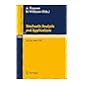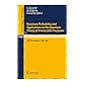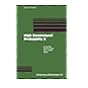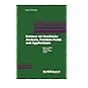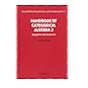No cover image available No cover image available 519.03 SWA Stochastic analysis and applications. Proceedings , Swansea, 1983. 519.03 VIL Quantum probability and applications to the quantum theory of irreversible processes. Proceedings, Villa Mondragone, 1982. 519.08 High dimensional probability II. Progress in Probability 47. 519.08 Encyclopedia of mathematics and its applications. Vol 12. Mathematical theory of entropy. 519.08 (Second) Seminar on stochastic analysis, random fields and applications, Centro Stefano Franscini, Ascona, 1996. Progress in Probability 45. 519.08 Handbook of categorical algebra 2. Categories and structures. Encyclopedia of Mathematics and its Applications 51. 519.08 Seminar on stochastic analysis, random fields and applications, Ascona, 1993. Progress in Probability 36.